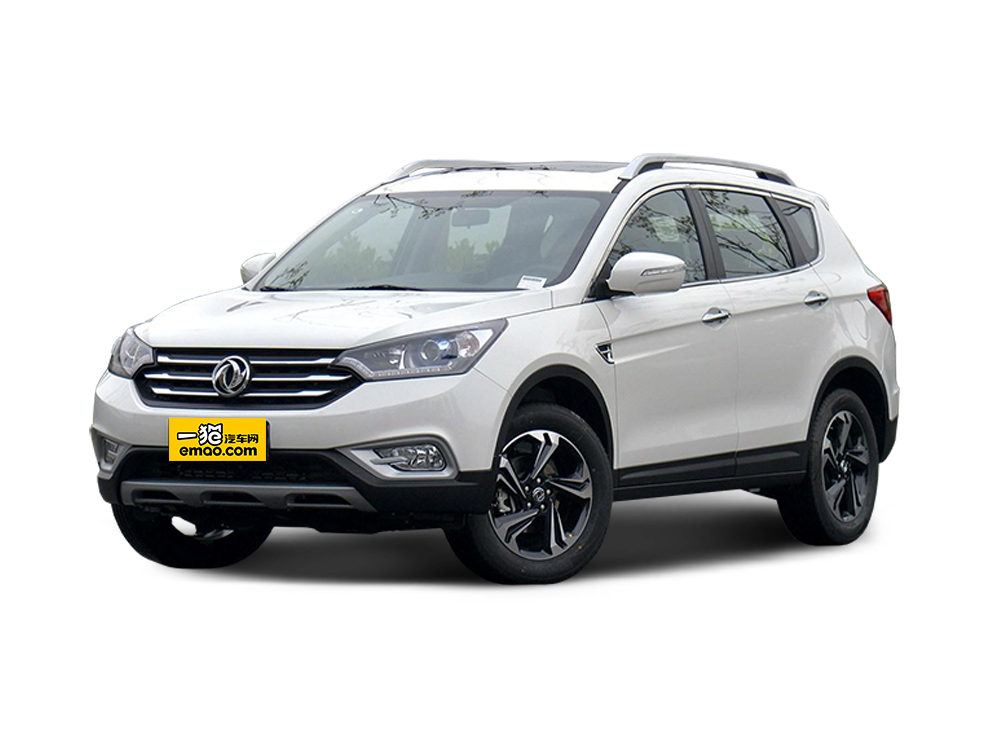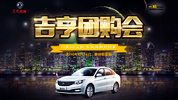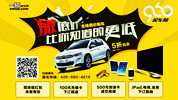【一猫汽车网武汉站】今年1-6月，东风汽车公司整体运营保持稳健，累计销售汽车192.69万辆，同比增长4.69%，销量排名稳居行业第二位。据7月最新公布的2016年《财富》世界500强榜单，东风汽车公司位居《财富》世界500强81位，较上年上升了28位，位居中国上榜企业第16位。下半年，东风公司将千方百计稳增长，确保完成400万辆销量目标，努力跑赢大市。

上半年，东风公司通过外拓市场，内强管理，以“详对标、补短板，强改革、抓机遇，提质量、增效益，起好步、开好局”为工作主线，坚决打好提质增效攻坚战，整体运营保持稳健，主要盈利指标均超额完成年度预算，市场销售指标同比稳定增长，为完成年度保增长目标奠定了基础。

产品结构优化促进东风销量增长。乘用车领域，自去年下半年起投放14款新品，SUV方面，东风日产新逍客、东风风神AX3、东风悦达起亚KX5、东风风光580得到用户青睐，产销两旺；轿车方面，东风本田新思域、东风日产新轩逸市场表现突出，销量超越上一代产品；MPV方面，东风小康风光、东风柳汽S500市场取得新突破。产品结构调整提升了东风公司市场竞争力。

自主合资双发力，东风乘用车市场表现稳定。自主乘用车快速增长，在新品的推动下东风乘用车公司、东风小康汽车有限公司销售均同比两位数增长。东风日产乘用车公司、东风本田汽车有限公司销售回升，尤其是东风本田在SUV拉动下销量高速增长。上半年，东风公司乘用车销售168.62万辆，同比增长5.17%，市场表现稳定。

新品投放加强，自主事业保持增长。上半年，东风公司累计销售自主品牌汽车65.4万辆，行业排名第三，同比增长5.03%，其中，自主品牌乘用车销售41.8万辆，同比增长6.94%，细分市场SUV增长较快、MPV增幅超过行业水平。上半年，自主商用车销售23.6万辆，同比增长1.80%。

当前，我国宏观经济发展进入新常态，呈现速度变化、结构优化、动力转换等三大特点，经济增长呈现L型走势。今后几年，总需求低迷和产能过剩并存的格局难以出现根本改变，L型走势将持续一段时间。经济增速回落，汽车行业增长也从过去两位数的高增长转向个位数的低增长或微增长，效益增长难度加大。

面对宏观经济的新变化，下半年东风公司将深入贯彻落实中央供给侧结构性改革和“三去一降一补”要求，把工作着力点放在提质增效、转型升级、结构调整、创新驱动上，推动发展量增质。

“跨越400万辆”，是东风公司下半年工作的重中之重。东风公司将积极把握市场机遇，进一步提振信心，咬定年初确定的400万辆目标，努力跑赢大市，持续深入落实“创新驱动、开放合作、主动主导、做强做优、共赢发展”等五大发展方针和“详对标、补短板，强改革、抓机遇，提质量、增效益，起好步、开好局”的工作要求，加快破解难题，既立足当前，着力防风险、稳增长，又着眼长远，着力打基础、增动力，确保“十三五”良好开局。

自主品牌和新能源汽车事业，一直是东风公司今后一个时期工作的重中之重。下半年将突出重点，力争取得新的突破。

自主乘用车方面，抓好机遇、乘势而上。将积极把握当前自主品牌汽车市场快速增长的机遇，多措并举确保必达目标，挑战更高目标，壮大自主品牌规模；进一步提升SUV、MPV销量，持续改善轿车销量，使更多已投放车型发挥应有价值。“十三五”时期，东风自主乘用车事业将通过推进资源协同、提升车型效率、培育明星产品等战略举措，不断提升东风自主乘用车的核心能力，使品牌影响力达到行业平均水平。

商用车方面，巩固向好趋势。中重型商用车业务，重点强化市场结构和产品结构调整，促进销量持续回升，加紧做好国五排放法规升级的应对措施。轻型商用车业务，坚持和巩固结构调整取得的良好态势，强化商品竞争力，实现良性发展。未来，东风商用车事业将从整车、动力总成、零部件水平事业等多个方面发力，重塑并保持东风商用车国内领先优势。

新能源汽车方面，加快把体制机制优化调整到位。进一步明确销售、示范运营、大客户管理、商业模式创新等业务的承担主体，理顺业务关系；大力推进电池等关键资源的掌控与协同；探索和创新商业模式，确保全年纯电动车2.3万辆的推广目标达成。“十三五”期间，东风公司将深入实施“583”计划（5项核心资源掌控、8项关键技术开发、3种商业模式创新），快速构建支撑东风新能源汽车发展的核心资源掌控体系，抢占新能源汽车制高点。

东风40多年的发展史就是一部变革求新、创新发展、转型调整的改革史。无论70年代、80年代、90年代、新世纪以来，东风都有许多敢为人先的大胆改革，让东风始终站在发展前列，走出了十堰大山，走出了湖北，走向了全国。东风公司的“芦席棚精神”、“马灯精神”，不仅仅是一种艰苦创业、自力更生的精神，也是一种敢为人先、勇于变革的精神。

为贯彻落实党的十八大、十八届三中全会关于全面深化国有企业改革的要求，东风公司成立了全面深化改革工作领导小组，进一步加强对改革工作的组织领导，致力于突破制约公司改革创新的瓶颈问题。目前，已明确了18个重要项目的改革思路和实施路径。

东风汽车公司董事长、党委书记竺延风说，东风公司必须抓住当前深化改革的“窗口期”，树立强烈的改革意识，加快摒除体制机制各种弊端，以焕发出强大的发展动力。

下半年和今后一个时期，东风公司将认真贯彻落实党中央、国务院和国资委的决策部署，围绕“瘦身健体、放活主体、体制机制创新”三条主线，因企施策，有力、有度、有效、有节奏地积极推进东风深化改革第一批重点课题与18个重点项目，着力在建立市场化机制、优化业务布局、解决历史遗留问题、提质增效、优化总部职能等五个方面寻求突破。

##### 相关资讯

|分享

•已阅！握爪
•我手滑为你点赞
•128个赞！
•不明觉厉
•阅后既醉
•有钱！任性
•照片太美，我不敢看
•干货！杠杠的
•高大上
•膜拜中！
•请接受我的膝盖
•猫哥/猫妹么么哒
•神吐槽
•我只笑笑不说话
•我想静静
•窒息
##### 资讯相关车系##### 热度排行• 阿斯顿·马丁
• 奥迪
• 阿尔法罗密欧
• ALPINA

• 巴博斯
• 宝骏
• 宝马
• 保时捷
• 北汽制造
• 奔驰
• 奔腾
• 本田
• 比亚迪
• 标致
• 别克
• 宾利
• 布加迪
• 北汽威旺
• 北京
• 北汽绅宝
• 北汽幻速
• 北汽新能源
• 宝沃
• 比速汽车
• 北汽道达

• 昌河
• 长安
• 长城
• 长安商用
• 成功汽车
• 长江EV
• 长安轻车型

• 大众
• 道奇
• 东风
• 东风风神
• 东风小康
• 东南
• DS
• 东风风行
• 东风风度
• 东风风光

• 法拉利
• 菲亚特
• 丰田
• 福迪
• 福特
• 福田汽车
• 福汽启腾
• 风诺

• GMC
• 广汽传祺
• 广汽吉奥
• 观致

• 哈飞
• 海格
• 海马
• 华泰
• 黄海
• 恒天
• 红旗
• 哈弗
• 华颂
• 华凯
• 华泰新能源
• 汉腾汽车

• Jeep
• 江淮
• 江铃
• 捷豹
• 金杯
• 九龙
• 吉利汽车
• 金旅
• 金龙
• 江铃集团轻汽
• 江铃集团新能源
• 君马汽车
• 捷途

• 开瑞
• 凯迪拉克
• 科尼赛克
• 克莱斯勒
• KTM
• 卡威
• 凯翼
• 康迪
• 康迪电动汽车集团

• 猎豹汽车
• 兰博基尼
• 劳斯莱斯
• 雷克萨斯
• 雷诺
• 理念
• 力帆
• 莲花汽车
• 林肯
• 铃木
• 陆风
• 路虎
• 路特斯
• 领志
• 领克
• 零跑汽车

• MG
• MINI
• 马自达
• 玛莎拉蒂
• 迈凯伦
• 摩根

• 纳智捷

• 讴歌
• 欧宝
• 欧朗
• 欧拉
• 欧尚汽车

• 帕加尼

• 奇瑞
• 启辰
• 起亚
• 前途
• 庆铃汽车

• 日产
• 荣威
• 瑞麒汽车
• 如虎
• 瑞驰

• 上汽大通
• smart
• 三菱
• 双环
• 双龙
• 斯巴鲁
• 斯柯达
• 萨博
• 思铭
• 赛麟
• SWM斯威汽车

• TESLA
• 泰卡特
• 腾势

• 威麟
• 威兹曼
• 沃尔沃
• 五菱汽车
• 五十铃
• 潍柴英致
• WEY
• 蔚来
• 威马汽车

• 现代
• 雪佛兰
• 雪铁龙
• 西雅特
• 新特汽车
• 小鹏汽车
• 新宝骏

• 野马汽车
• 一汽
• 依维柯
• 英菲尼迪
• 永源
• 驭胜

• 中华
• 中兴
• 众泰
• 知豆
• 之诺
• 正道汽车
• A
• B
• C
• D
• E
• F
• G
• H
• I
• J
• K
• L
• M
• N
• O
• P
• Q
• R
• S
• T
• U
• V
• W
• X
• Y
• Z

• 阿斯顿·马丁
• 奥迪
• 阿尔法罗密欧
• ALPINA

• 巴博斯
• 宝骏
• 宝马
• 保时捷
• 北汽制造
• 奔驰
• 奔腾
• 本田
• 比亚迪
• 标致
• 别克
• 宾利
• 布加迪
• 北汽威旺
• 北京
• 北汽绅宝
• 北汽幻速
• 北汽新能源
• 宝沃
• 比速汽车
• 北汽道达

• 昌河
• 长安
• 长城
• 长安商用
• 成功汽车
• 长江EV
• 长安轻车型

• 大众
• 道奇
• 东风
• 东风风神
• 东风小康
• 东南
• DS
• 东风风行
• 东风风度
• 东风风光

• 法拉利
• 菲亚特
• 丰田
• 福迪
• 福特
• 福田汽车
• 福汽启腾
• 风诺

• GMC
• 广汽传祺
• 广汽吉奥
• 观致

• 哈飞
• 海格
• 海马
• 华泰
• 黄海
• 恒天
• 红旗
• 哈弗
• 华颂
• 华凯
• 华泰新能源
• 汉腾汽车

• Jeep
• 江淮
• 江铃
• 捷豹
• 金杯
• 九龙
• 吉利汽车
• 金旅
• 金龙
• 江铃集团轻汽
• 江铃集团新能源
• 君马汽车
• 捷途

• 开瑞
• 凯迪拉克
• 科尼赛克
• 克莱斯勒
• KTM
• 卡威
• 凯翼
• 康迪
• 康迪电动汽车集团

• 猎豹汽车
• 兰博基尼
• 劳斯莱斯
• 雷克萨斯
• 雷诺
• 理念
• 力帆
• 莲花汽车
• 林肯
• 铃木
• 陆风
• 路虎
• 路特斯
• 领志
• 领克
• 零跑汽车

• MG
• MINI
• 马自达
• 玛莎拉蒂
• 迈凯伦
• 摩根

• 纳智捷

• 讴歌
• 欧宝
• 欧朗
• 欧拉
• 欧尚汽车

• 帕加尼

• 奇瑞
• 启辰
• 起亚
• 前途
• 庆铃汽车

• 日产
• 荣威
• 瑞麒汽车
• 如虎
• 瑞驰

• 上汽大通
• smart
• 三菱
• 双环
• 双龙
• 斯巴鲁
• 斯柯达
• 萨博
• 思铭
• 赛麟
• SWM斯威汽车

• TESLA
• 泰卡特
• 腾势

• 威麟
• 威兹曼
• 沃尔沃
• 五菱汽车
• 五十铃
• 潍柴英致
• WEY
• 蔚来
• 威马汽车

• 现代
• 雪佛兰
• 雪铁龙
• 西雅特
• 新特汽车
• 小鹏汽车
• 新宝骏

• 野马汽车
• 一汽
• 依维柯
• 英菲尼迪
• 永源
• 驭胜

• 中华
• 中兴
• 众泰
• 知豆
• 之诺
• 正道汽车
• A
• B
• C
• D
• E
• F
• G
• H
• I
• J
• K
• L
• M
• N
• O
• P
• Q
• R
• S
• T
• U
• V
• W
• X
• Y
• Z

• 阿斯顿·马丁
• 奥迪
• 阿尔法罗密欧
• ALPINA

• 巴博斯
• 宝骏
• 宝马
• 保时捷
• 北汽制造
• 奔驰
• 奔腾
• 本田
• 比亚迪
• 标致
• 别克
• 宾利
• 布加迪
• 北汽威旺
• 北京
• 北汽绅宝
• 北汽幻速
• 北汽新能源
• 宝沃
• 比速汽车
• 北汽道达

• 昌河
• 长安
• 长城
• 长安商用
• 成功汽车
• 长江EV
• 长安轻车型

• 大众
• 道奇
• 东风
• 东风风神
• 东风小康
• 东南
• DS
• 东风风行
• 东风风度
• 东风风光

• 法拉利
• 菲亚特
• 丰田
• 福迪
• 福特
• 福田汽车
• 福汽启腾
• 风诺

• GMC
• 广汽传祺
• 广汽吉奥
• 观致

• 哈飞
• 海格
• 海马
• 华泰
• 黄海
• 恒天
• 红旗
• 哈弗
• 华颂
• 华凯
• 华泰新能源
• 汉腾汽车

• Jeep
• 江淮
• 江铃
• 捷豹
• 金杯
• 九龙
• 吉利汽车
• 金旅
• 金龙
• 江铃集团轻汽
• 江铃集团新能源
• 君马汽车
• 捷途

• 开瑞
• 凯迪拉克
• 科尼赛克
• 克莱斯勒
• KTM
• 卡威
• 凯翼
• 康迪
• 康迪电动汽车集团

• 猎豹汽车
• 兰博基尼
• 劳斯莱斯
• 雷克萨斯
• 雷诺
• 理念
• 力帆
• 莲花汽车
• 林肯
• 铃木
• 陆风
• 路虎
• 路特斯
• 领志
• 领克
• 零跑汽车

• MG
• MINI
• 马自达
• 玛莎拉蒂
• 迈凯伦
• 摩根

• 纳智捷

• 讴歌
• 欧宝
• 欧朗
• 欧拉
• 欧尚汽车

• 帕加尼

• 奇瑞
• 启辰
• 起亚
• 前途
• 庆铃汽车

• 日产
• 荣威
• 瑞麒汽车
• 如虎
• 瑞驰

• 上汽大通
• smart
• 三菱
• 双环
• 双龙
• 斯巴鲁
• 斯柯达
• 萨博
• 思铭
• 赛麟
• SWM斯威汽车

• TESLA
• 泰卡特
• 腾势

• 威麟
• 威兹曼
• 沃尔沃
• 五菱汽车
• 五十铃
• 潍柴英致
• WEY
• 蔚来
• 威马汽车

• 现代
• 雪佛兰
• 雪铁龙
• 西雅特
• 新特汽车
• 小鹏汽车
• 新宝骏

• 野马汽车
• 一汽
• 依维柯
• 英菲尼迪
• 永源
• 驭胜

• 中华
• 中兴
• 众泰
• 知豆
• 之诺
• 正道汽车
• A
• B
• C
• D
• E
• F
• G
• H
• I
• J
• K
• L
• M
• N
• O
• P
• Q
• R
• S
• T
• U
• V
• W
• X
• Y
• Z